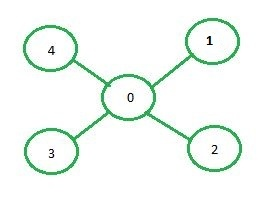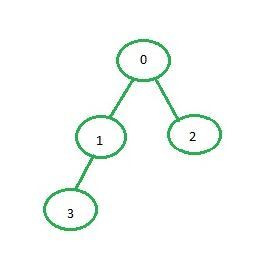# Implementation of DFS using adjacency matrix

Adjacency matrix representation: In adjacency matrix representation of a graph, the matrix mat[][] of size n*n (where n is the number of vertices) will represent the edges of the graph where mat[i][j] = 1 represents that there is an edge between the vertices i and j while mat[i][i] = 0 represents that there is no edge between the vertices i and j.Below is the adjacency matrix representation of the graph shown in the above image:

```   0 1 2 3 4
0  0 1 1 1 1
1  1 0 0 0 0
2  1 0 0 0 0
3  1 0 0 0 0
4  1 0 0 0 0
```

Examples:

```Input: source = 0Output: 0 1 3 2

Input: source = 0Output: 0 1 2 3 4
```

## Recommended: Please try your approach on {IDE} first, before moving on to the solution.

Approach:

• Create a matrix of size n*n where every element is 0 representing there is no edge in the graph.
• Now, for every edge of the graph between the vertices i and j set mat[i][j] = 1.
• After the adjacency matrix has been created and filled, call the recursive function for the source i.e. vertex 0 that will recursively call the same function for all the vertices adjacent to it.
• Also, keep an array to keep track of the visited vertices i.e. visited[i] = true represents that vertex i has been been visited before and the DFS function for some already visited node need not be called.

Below is the implementation of the above approach:

 `// C++ implementation of the approach ` `#include ` `using` `namespace` `std; ` ` `  `class` `Graph { ` ` `  `    ``// Number of vertex ` `    ``int` `v; ` ` `  `    ``// Number of edges ` `    ``int` `e; ` ` `  `    ``// Adjacency matrix ` `    ``int``** adj; ` ` `  `public``: ` `    ``// To create the initial adjacency matrix ` `    ``Graph(``int` `v, ``int` `e); ` ` `  `    ``// Function to insert a new edge ` `    ``void` `addEdge(``int` `start, ``int` `e); ` ` `  `    ``// Function to display the DFS traversal ` `    ``void` `DFS(``int` `start, vector<``bool``>& visited); ` `}; ` ` `  `// Function to fill the empty adjacency matrix ` `Graph::Graph(``int` `v, ``int` `e) ` `{ ` `    ``this``->v = v; ` `    ``this``->e = e; ` `    ``adj = ``new` `int``*[v]; ` `    ``for` `(``int` `row = 0; row < v; row++) { ` `        ``adj[row] = ``new` `int``[v]; ` `        ``for` `(``int` `column = 0; column < v; column++) { ` `            ``adj[row][column] = 0; ` `        ``} ` `    ``} ` `} ` ` `  `// Function to add an edge to the graph ` `void` `Graph::addEdge(``int` `start, ``int` `e) ` `{ ` ` `  `    ``// Considering a bidirectional edge ` `    ``adj[start][e] = 1; ` `    ``adj[e][start] = 1; ` `} ` ` `  `// Function to perform DFS on the graph ` `void` `Graph::DFS(``int` `start, vector<``bool``>& visited) ` `{ ` ` `  `    ``// Print the current node ` `    ``cout << start << ``" "``; ` ` `  `    ``// Set current node as visited ` `    ``visited[start] = ``true``; ` ` `  `    ``// For every node of the graph ` `    ``for` `(``int` `i = 0; i < v; i++) { ` ` `  `        ``// If some node is adjacent to the current node ` `        ``// and it has not already been visited ` `        ``if` `(adj[start][i] == 1 && (!visited[i])) { ` `            ``DFS(i, visited); ` `        ``} ` `    ``} ` `} ` ` `  `// Driver code ` `int` `main() ` `{ ` `    ``int` `v = 5, e = 4; ` ` `  `    ``// Create the graph ` `    ``Graph G(v, e); ` `    ``G.addEdge(0, 1); ` `    ``G.addEdge(0, 2); ` `    ``G.addEdge(0, 3); ` `    ``G.addEdge(0, 4); ` ` `  `    ``// Visited vector to so that ` `    ``// a vertex is not visited more than once ` `    ``// Initializing the vector to false as no ` `    ``// vertex is visited at the beginning ` `    ``vector<``bool``> visited(v, ``false``); ` ` `  `    ``// Perform DFS ` `    ``G.DFS(0, visited); ` `} `

Output:

```0 1 2 3 4
```

Don’t stop now and take your learning to the next level. Learn all the important concepts of Data Structures and Algorithms with the help of the most trusted course: DSA Self Paced. Become industry ready at a student-friendly price.

My Personal Notes arrow_drop_upCheck out this Author's contributed articles.

If you like GeeksforGeeks and would like to contribute, you can also write an article using contribute.geeksforgeeks.org or mail your article to contribute@geeksforgeeks.org. See your article appearing on the GeeksforGeeks main page and help other Geeks.

Please Improve this article if you find anything incorrect by clicking on the "Improve Article" button below.

Improved By : deepesh08